# Electronics and Communication Engineering - Automatic Control Systems

Exercise : Automatic Control Systems - Section 1
21.
In the given figure the input frequency is such that R << Xc, then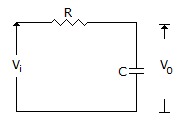v0 lags vi by 90°
v0 and vi are in phase
either (b) or (c)
Explanation:

Voltage across capacitor lags by 90°.

22.
In the given figure, of potentiometer V0 = Vi (R0/Ri) only when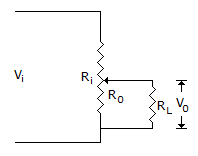RL = 0
RL = ∞
RL is neither low nor high
RL is low but not zero
Explanation:

If RL is not infinite, the voltage ratio of potentiometer depends on the value of RL. This is called loading effect.

23.
A unity feedback system has open loop transfer function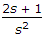The closed loop transfer function is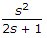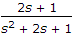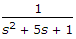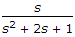Explanation: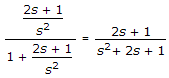.

24.
Bellows converts
pressure difference into displacement
pressure difference into voltage
displacement into pressure difference
either (a) or (c)
Explanation:

To measure pressure, it is convenient to convert pressure into displacement and then use a displacement transducer.

25.

Assertion (A): The steady state response, of a stable, linear, time invariant system, to sinusoidal input depends on initial conditions.

Reason (R): Frequency response, in steady state, is obtained by replacing s in the transfer function by jω

Both A and R are correct and R is correct explanation of A
Both A and R are correct but R is not correct explanation of A
A is correct but R is wrong
R is correct but A is wrong